Science, Maths & Technology

### Become an OU student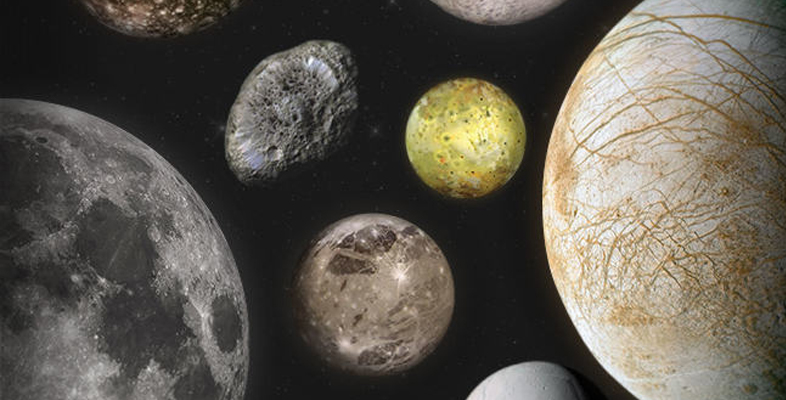Moons of our Solar System

Start this free course now. Just create an account and sign in. Enrol and complete the course for a free statement of participation or digital badge if available.

# 3.4 Surface gravity

The strength of surface gravity on the Earth (measured as the rate at which a falling object accelerates) is 9.8 m s−2 (where m s−2 stands for metres per second per second, meaning the rate at which the speed of a falling object increases).

Read the following information and then try Activity 3, where you are asked to match the correct value of surface gravity to different moons. Feel your way by making intelligent guesses – we don’t expect you to work out the value from first principles.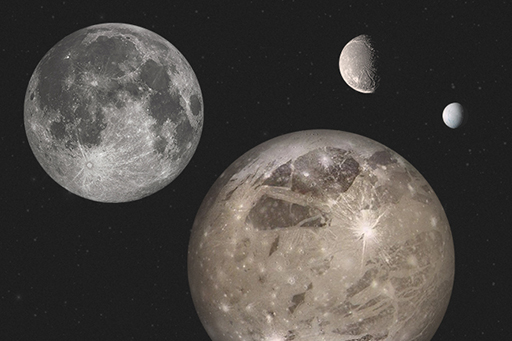Figure 38 The Moon, Ganymede, Ariel and Enceladus, shown at correct relative scale.
• The Moon is a rocky body smaller than the Earth, whose surface gravity is about one-sixth of the Earth’s and whose diameter is 3474 km.
• Ganymede is an icy moon of Jupiter whose diameter is 5262 km. Ice is less dense than rock.
• Enceladus is an icy moon of Saturn whose diameter is 504 km.
• Ariel is an icy moon of Uranus whose diameter is 1158 km.

## Activity 3 Surface gravity – what do you know?

Timing: Allow approximately 15 minutes.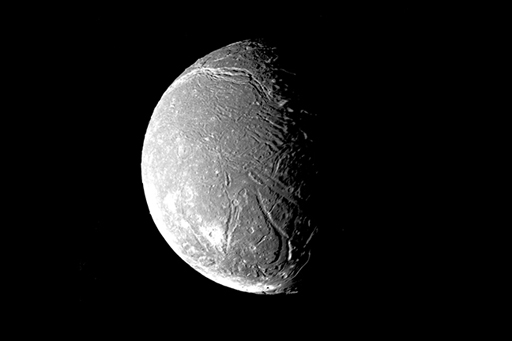Figure 39 Ariel.

What is the surface gravity on this moon?

a.

0.114 m s-2

b.

1.428 m s-2

c.

0.270 m s-2

d.

1.622 m s-2

The correct answer is c.

c.

Well done.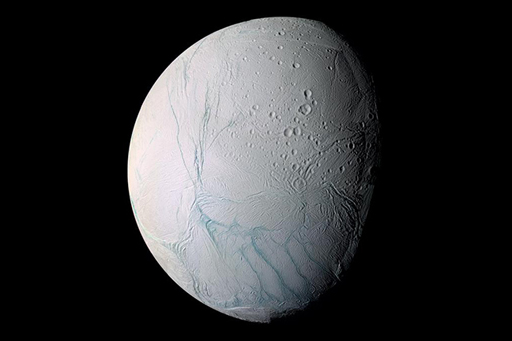What is the surface gravity on this moon?

a.

0.114 m s-2

b.

1.428 m s-2

c.

0.270 m s-2

d.

1.622 m s-2

The correct answer is a.

a.

Well done. The surface gravity on Enceladus is not much more than one-hundredth of the Earth’s.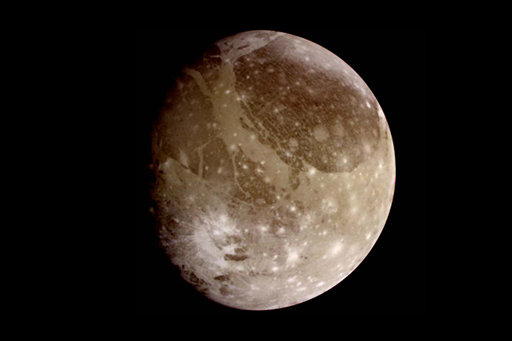Figure 41 Ganymede.

What is the surface gravity on this moon?

a.

0.114 m s-2

b.

1.428 m s-2

c.

0.270 m s-2

d.

1.622 m s-2

The correct answer is b.

b.

Well done.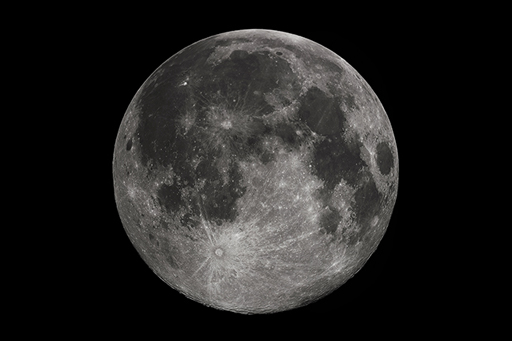Figure 42 The Moon

What is the surface gravity on the Moon?

a.

0.114 m s-2

b.

1.428 m s-2

c.

0.270 m s-2

d.

1.622 m s-2

The correct answer is d.

d.

Well done. Although Ganymede is bigger (and has more mass) than the Moon, it is less dense. The net result is that its surface gravity is slightly less than the Moon’s.

If you wanted to look into this further, you might find the following link of interest: Detection of new craters on images [Tip: hold Ctrl and click a link to open it in a new tab. (Hide tip)] . 222 new craters (10 m to 43 m in size) found by comparing high-resolution images from NASA’s Lunar Reconnaissance Orbiter. Short article and animation.